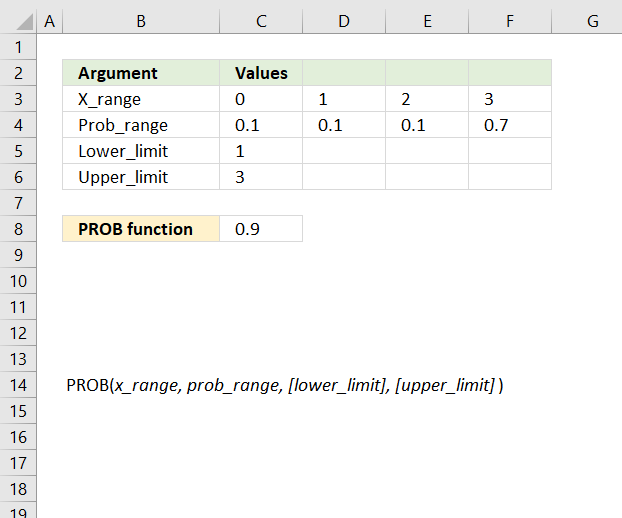Author: Oscar Cronquist Article last updated on August 24, 2018The PROB function calculates the probability that values in a range are between a given lower and upper limit. Probability is a value that shows how likely something is going to happen.

Formula in cell C8:

=PROB(C3:F3,C4:F4,C5,C6)

### Excel Function Syntax

PROB(x_range, prob_range, [lower_limit], [upper_limit])

### Arguments

 X_range Required. The bond's settlement date, in other words, the date a buyer purchases a security. Prob_range Required. The bond's maturity date, in other words, when it expires. Lower_limit Optional. The lower bound on the range for which you want to know the probability. Upper_limit Optional. The upper bound on the range for which you want to know the probability. If omitted the PROB function returns the probability of being equal to the lower_limit.